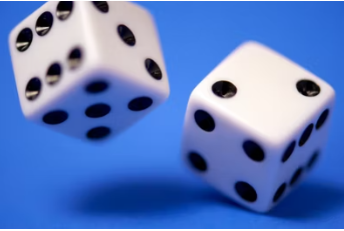## Board Games

Consider you are playing a board game, and it's your turn to throw two 6-sided dice. What is the probability you roll a 11 or less?Hint
There is only one scenario (6 and 6) that does not fall into the desired range.
Hint 2
There are a total of 36 possible outcomes for the two dice $$(6\times6=36)$$ .
There is only one scenario (6 and 6) that does not fall into the desired range. There are also 36 possible outcomes for the two dice $$(6\times6=36)$$ . Therefore, there is a 35/36 chance the sum will be 11 or less.
$$\frac{35}{36}=0.9721\:probability$$$$$\frac{35}{36}=0.9721\:probability$$$